DC Amplifier for Pyranometer

Wichit Sirichote, kswichit@kmitl.ac.th

Simple experiment demonstrates the use of Minilogger for recording the amount of sun energy received everyday.

The study program for 3rd Year Applied Physics students has a course on Measurement Laboratory I. The students must attend for 10 labs. I have three labs, 1) Ultrasonic Measurement: to measure sound velocity in the water with pulse-echo-overlap, 2) THDV Measurement: to study how 3rd harmonic appears in AC supply and how to measurement such distortion and 3) Solar Radiation Measurement: to study how to measure daily insolation and to find the system efficiency of the utility backup PV system. This led me to develop the signal conditioning circuit to amplify the EMF produced by pyranometer.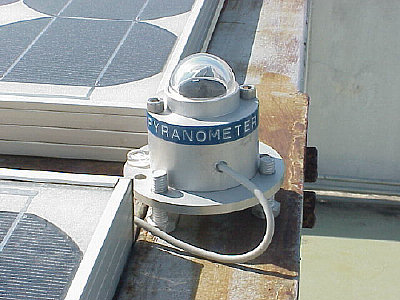Figure 1: Pyranometer for total radiation measurement.

The EMF is 14uV/Wm-2. The analog input of minilogger accepts +/-400.0mV with 100 uV sensitivity. So we will need DC amplifier to provide DC gain for input of 14uV to 100uV or x7.14.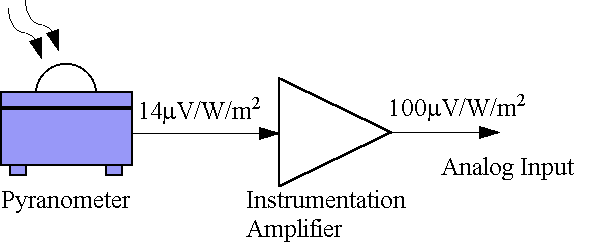Figure 2: Precision DC amplifier using INA101 Instrumentation Amplifier.

The circuit is simple, we just have Rg tie to pin 4,5 and 10,11 for DC gain adjustment and have decoupling caps with 0.1uF disceramic parallel by 4.7uF tantalum capacitor. Vout can tie to input of minilogger easily.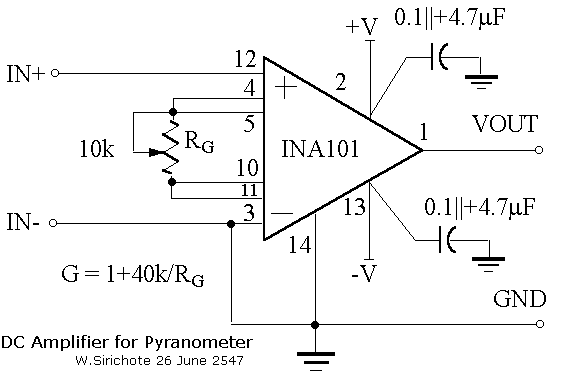Figure 3: Schematic of DC Amplifer.

Now the minilogger can start/stop record with preset time. I tested with start at 6:00 and stop at 18:00 with 1 minute sampling interval. The total number of record is 720. Figure 4 shows plot of insolation along 6:00 to 18:00. The total insolation computed by summing all records then divided by 60 gives 5403Wh/m2 per day.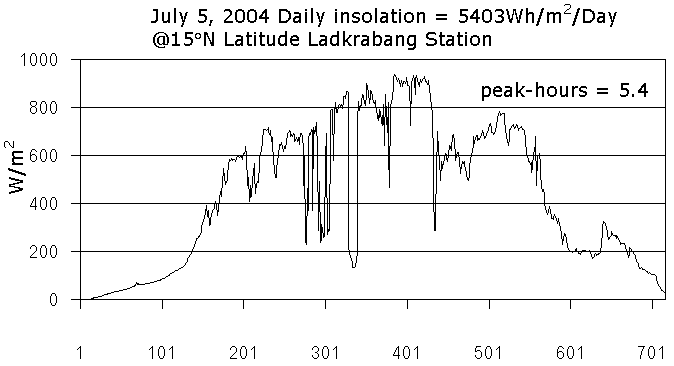Figure 4: Using Excel plots insolation vs time from 6:00 to 18:00.

My solar lab has the utility backup Photovoltaic power system. The PV array is 3kWp made with Silicon solar cell. The operation of power conditioning unit, PCU and PV array emulates AC negative load. With a bi-directional energy meter, and no AC positive load connected, the meter will spin backward. The total AC energy in kWh will be recorded from 6:00 to 18:00.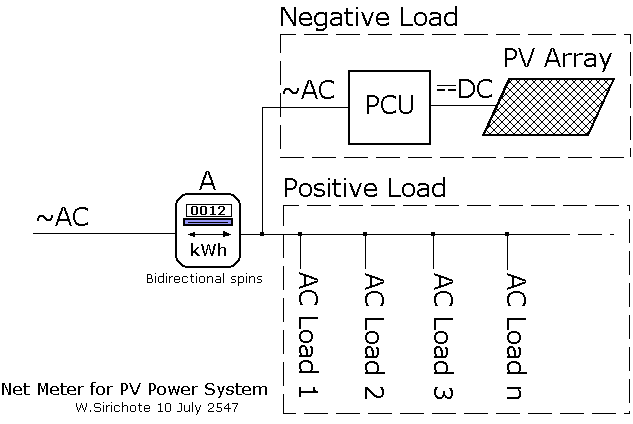Figure 5: Net Metering PV Power System.

We can find the efficiency of the system by equation shown below. The output AC energy will be divided by sun energy. Total area of PV array (A) is 19.469 m2 (two strings).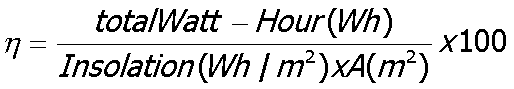last updated: July 11, 2004